# JAC Class 10 Maths Solutions Chapter 14 Statistics Ex 14.3

Jharkhand Board JAC Class 10 Maths Solutions Chapter 14 Statistics Ex 14.3 Textbook Exercise Questions and Answers.

## JAC Board Class 10 Maths Solutions Chapter 14 Statistics Exercise 14.3

Question 1.
The following frequency distribution gives the monthly consumption of electricity of 68 consumers of a locality. Find the media, mean and mode of the data and compare them.

 Monthly consumption (in units) No. of consumers 65 – 85 4 85 – 105 5 105 – 125 13 125 – 145 20 145 – 165 14 165 – 185 8 185 – 205 4

Solution: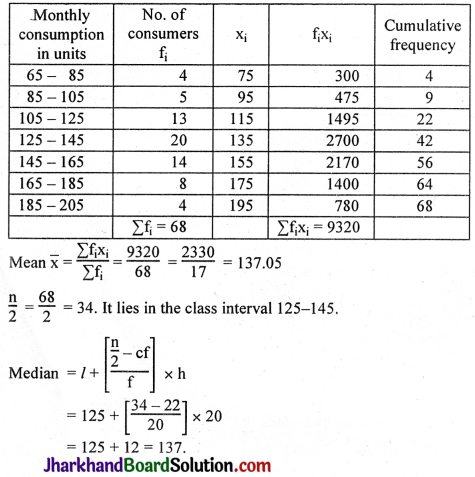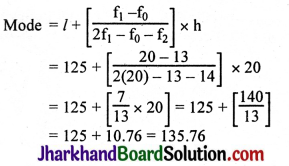Mean is 137 units.
Median is 137 units.
Mode is 135.76 units.
The three measures are approximately the same.Question 2.
If the median of the distribution given below is 28.5, find the values of x and y.

 Class interval Frequency 0 – 10 5 10 – 20 x 20 – 30 20 30 – 40 15 40 – 50 y 50 – 60 5 Total 60

Solution:

 Class interval Frequency Cumulative frequency 0 – 10 5 5 10 – 20 x 5 + x 20 – 30 20 25 + x 30 – 40 15 40 + x 40 – 50 y 40 + x + y 50 – 60 5 45 + x + y 60

n = 60, 45 + x + y = 60
x + y = 60 – 45
x + y = 15
The median is 28.5. It lies in the class interval 20 – 30.
∴ l = 20, f = 20, cf = 5 + x, h = 10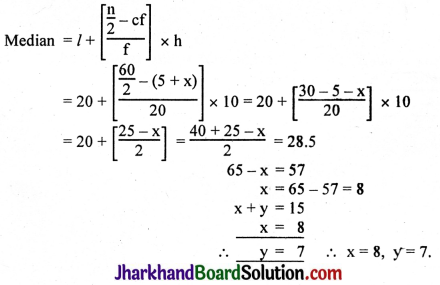Question 3.
A life insurance agent found the following data for distribution of ages of 100 policyholders. Calculate the median age, if policies are given only to persons having age 18 years onwards but less than 60 years.

 Age (in years) No. of policyholders Below 20 2 Below 25 6 Below 30 24 Below 35 45 Below 40 78 Below 45 89 Below 50 92 Below 55 98 Below 60 100

Solution:

 Class interval No. of policyholders c.f. Below 20 2 2 20 – 25 4 6 25 – 30 18 24 30 – 35 21 45 35 – 40 33 78 40 – 45 11 89 45 – 50 3 92 50 – 55 6 98 55 – 60 2 100 n = 100 $$\frac{n}{2}$$ = 50

l = 35, $$\frac{n}{2}$$ = 50, cf = 45, f = 33, h = 5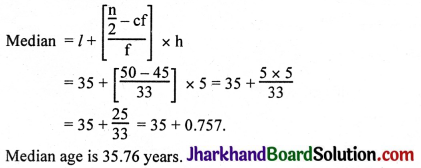Question 4.
The lengths of 40 leaves of a plant are measured correct to the nearest millimetre, and the data obtained is represented in the following table:

 Length (in mm) Number of leaves 118 – 126 3 127 – 135 5 136 – 144 9 145 – 153 12 154 – 162 5 163 – 171 4 172 – 180 2

Find the median length of the leaves.
(Hint: The data need to be converted to continuous classes for finding the median, since the formula assumes continuous classes. The classes then change to 117.5 – 126.5, 126.5 – 135.5, ….., 171.5 – 180.5).
Solution:
The data have to be converted to continuous classes for finding the median since the formula. assumes continuous classes.

 Class interval No. of leaves Cumulative frequency (cf) 117.5 – 126.5 3 3 126.5 – 135.5 5 8 135.5 – 144.5 9 17 144.5 – 153.5 12 29 153.5 – 162.5 5 34 162.5 – 171.5 4 38 171.5 – 180.5 2 40

n = 40, $$\frac{n}{2}$$ = 20
The median lies in the class interval 144.5 – 153.5.
l = 144.5, $$\frac{\mathrm{n}}{2}=\frac{40}{2}$$ = 20, cf = 17, f = 12, h = 9.
Median = l + $$\left[\frac{\frac{n}{2}-c f}{f}\right]$$ × h
= 144.5 + $$\left[\frac{20-17}{12} \times 9\right]$$
= 144.5 + $$\left[\frac{27}{12}\right]$$
= 144.5 + 2.25
= 146.75 mm.

Question 5.
The following table gives the distribution of the lifetime of 400 neon lamps:

 Lifetime (in hours) Number of lamps 1500 – 2000 14 2000 – 2500 56 2500 – 3000 60 3000 – 3500 86 3500 – 4000 74 4000 – 4500 62 4500 – 5000 48

Find the median lifetime of a lamp.
Solution:

 Lifetime in hours (CI) No. of lamps (l) Cumulative frequency 1500 – 2000 14 14 2000 – 2500 56 70 2500 – 3000 60 130 3000 – 3500 86 216 3500 – 4000 74 290 4000 – 4500 62 352 4500 – 5000 48 400

The median lies in the class interval 3000 – 3500.
$$\frac{\mathrm{n}}{2}=\frac{400}{2}=200$$
l = 3000, $$\frac{n}{2}$$ = 200, cf = 130, f = 86, h = 500.
Median = l + $$\left[\frac{\frac{\mathrm{n}}{2}-\mathrm{cf}}{\mathrm{f}}\right]$$ × h
= 3000 + $$\left[\frac{200-130}{86}\right]$$ × 500
= 3000 + $$\left[\frac{70}{86}\right]$$ × 500
= 3000 + $$\frac{35000}{86}$$
= 3000 + 406.976
Median life of a lamp is 3406.98 hours.Question 6.
100 surnames were randomly picked up from a local telephone directory and the frequency distribution of the number of letters in the English alphabet in the surnames was obtained as follows:

 No. of letters No. of surnames 1 – 4 6 4 – 7 30 7 – 10 40 10 – 13 16 13 – 16 4 16 – 19 4

Determine the median number of letters in the surnames. Find the mean number of letters in the surnames. Also, find the modal size of the surnames.
Solution: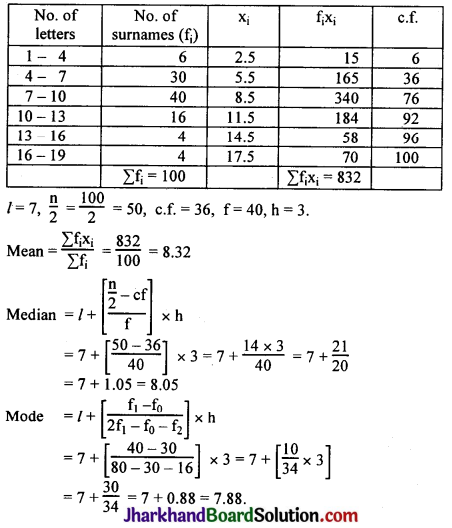Hence the modal size of the surnames is 7.88.Question 7.
The distribution below gives the weights of 30 students of a class. Find the median weight of the students.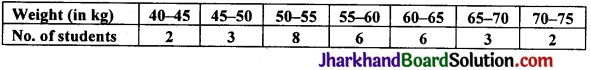Solution:

 Weight in kg. No. of students Cumulative frequency (c.f.) 40 – 45 2 2 45 – 50 3 5 50 – 55 8 13 55 – 60 6 19 60 – 65 6 25 65 – 70 3 28 70 – 75 2 30

$$\frac{n}{2}$$ = 15
The median lies in the class 55 – 60.
l = 55, $$\frac{n}{2}$$ = 15, c.f. = 13, f = 6, h = 5.
Median = l + $$\frac{1}{2}$$ × h
= 55 + $$\left[\frac{15-13}{6}\right]$$ × 5
= 55 + $$\frac{2}{6}$$ × 5
= 55 + $$\frac{5}{3}$$
= 55 + 1.666 = 56.666
∴ Median = 56.67 kg.
Hence, the median weight of the students is 56.67 kg.